Probability Basics
 Home > Lessons > Probability Basics Search | Updated May 16th, 2021
Introduction

In this section of MATHguide, you will learn how to calculate basic probability problems. These topics will be presented here:

 Understanding Probabilities Probability is the quantification of determining the likelihood of an event. What does probability mean to car manufacturers?     Car manufacturers extend new car customers warranties. These warranties protect the owner in case something breaks, like non-wear and tear items, such as: transmission, engine, and electrical systems. If one of these major systems gets damaged while a car is under warranty, the car will be fixed for free. Car buyers like this protection because it gives them a sense of comfort buying a new automobile.It is therefore very important that car companies know how long their car parts will last. They need to know the likelihood of a part failing before a warranty expires. If the part is too likely to fail before the expiration of the warranty, it will cost the car company money to repair the part and it may also make the customer feel hesitant to buy a car from the manufacturer.     These probabilities are important in several industries other than the automotive industry. An entire branch of mathematics, called actuarial science, exists to calculate risk for many industries. Actuaries study risk for insurance, finance, and government programs involving mortality. retirement, and predicting the future. Actuaries are respected and well paid. Prerequisite Knowledge The lesson that follows demands you already know the following lessons. If you are unfamiliar with these lessons, it is strongly suggested you review them first before proceeding.esson: Fractions, Decimals, and Percentsesson: Percent Word Problems Probability Formula The calculation for probability involves counting events.     The probability of a certain event, called event A, is written as P(A). Probability is then calculated as follows.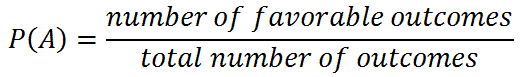The examples below will run through a variety of circumstances that will demonstrate how to use this formula. Spinner Problems There are many games that use a spinner: like Twister, Life, and Mousetrap. A player spins the arrow on a spinner, the spinner goes wildly in circles, and then the arrow lands on a space. We will use the spinner found below to do several examples using the formula for probability.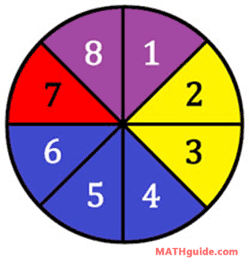Example 1: Calculate P(spinning 2). There are eight possible outcomes, {1,2,3,4,5,6,7,8} if we only look at numbers and not colors. The number two is one of those numbers. So, the probability is...Example 2: Calculate P(spinning a number < 3). There are two numbers less than 3, namely {1,2}. There are eight possible outcomes {1,2,3,4,5,6,7,8}. So, the probability is...Example 3: Calculate P(an even blue number). Favorable outcomes will be both blue and even numbers. The blue numbers that are even are {4,6}. So, there are 2 favorable outcomes out of 8 possible outcomes.Try our quizmaster to see if you fully understand this lesson.uiz: Probability: Spinner Problems Marble Bag Problems Probability problems would not be complete if we did not look at a bag of marbles. Marble bag problems are typically found in math textbooks and they do a great job demonstrating the need to be an accurate counter. We will use the marble bag below to demonstrate how marble bag problems work using the formula for probability.Example 1: Calculate P(pulling a red marble). There are three red marbles. There are a total of ten marbles in the bag. The probability is therefore...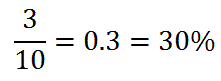Example 2: Calculate P(pulling a green marble). There are four green marbles. There are a total of ten marbles in the bag. The probability is therefore...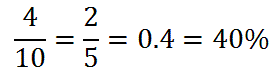Example 3: Calculate P(pulling a yellow, blue, or red marble). There is 1 yellow, 2 blue, and 3 red marbles. We have 6 marbles that can be pulled that are considered favorable outcomes. There are a total of ten marbles in the bag. The probability is therefore...Watch our video and try our quizmaster to see if you fully understand this lesson.ideo: Probability: Marble Bag Problemsuiz: Probability: Marble Bag Problems Dice Problems Most board games include dice, which means most people have seen 6-sided dice, like these.When we roll a 6-sided die, the possible outcomes are {1,2,3,4,5,6}. Calculating probabilities using the formula for probability with a single die are relatively simple because they are similar to spinner problems. However, let us look at what happens when we roll two dice and record the outcomes. Let this table reveal the possible outcomes.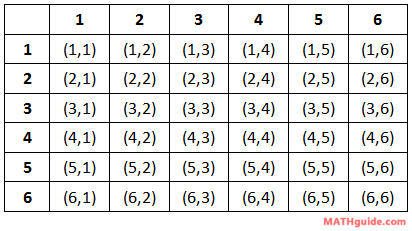We will use this table to determine the probability problems below. Example 1: Calculate P(rolling doubles). There are six cases when doubles are rolled, which are highlighted below.These are the cases as a list: {(1,1), (2,2), (3,3), (4,4), (5,5), (6,6)}. There are a total of 36 possible outcomes. So, the probability is...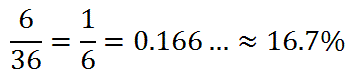Example 2: Calculate P(not rolling any 5s). If we cannot roll any fives, let's look at the cases that involve fives, highlighted below.Counting them reveals eleven cases. Keeping in mind there are a total of 36 possible outcomes and we cannot consider these highlighted 11 cases, there are therefore 36 - 11, or 25 favorable outcomes. This means our probability is...Example 3: Calculate P(rolling a sum < 6). We have to re-examine the die-rolling table so that it reflects dice sums, as follows.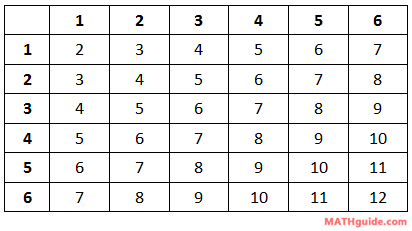Let's see which sums are less than six.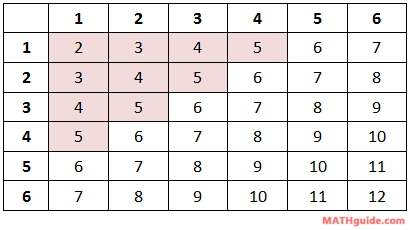There are ten highlighted sums found above. So, that means our probability is...Use these resources to solidify your understanding of dice problems.ideo: Probability of Rolling Diceuiz: Probability: Dice Problems Videos Try our instructional videos to help learn this material.ideo: Probability of Rolling Diceideo: Probability: Marble Bag Problemsideo: The Monty Hall Problem: A Game Show Probability Quizmasters Try our interactive quiz to determine if you understand the lessons above.uiz: Probability: Spinner Problemsuiz: Probability: Marble Bag Problemsuiz: Probability: Dice Problems     The quiz below is actually an interactive experiment involving three cars that compete in a professional drag race. Use your knowledge of probability to determine if a certain car has an advantage over the others if they compete two at a time in elimination.uiz: Drag Race Related Lessons Try these lessons, which are closely related to the lessons above.esson: Basic Countingesson: Complex Countingesson: Placement Countingesson: Monty Hall Problem